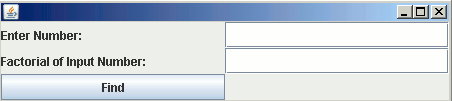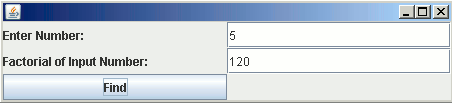# Java Swing Compute Factorial

In this section, we are going to find the factorial of a given number.

In this section, we are going to find the factorial of a given number.

### Java Swing Compute Factorial

In this section, we are going to find the factorial of a given number. Here an user is allowed to enter a number into the text field whose factorial is to be determined. On pressing the button the value of the text field is firstly converted into integer and then processed to find its factorial. The result will get displayed in another text field.

Here is the code:

```import java.awt.*;
import javax.swing.*;
import java.awt.event.*;

class FactorialExample extends JFrame {
JTextField t1, t2;

FactorialExample() {
JLabel l1 = new JLabel("Enter Number: ");
JLabel l2 = new JLabel("Factorial of Input Number: ");
t1 = new JTextField(20);
t2 = new JTextField(20);
JPanel p = new JPanel(new GridLayout(3, 2));
JButton b = new JButton("Find");
public void actionPerformed(ActionEvent e) {
String number = t1.getText();
int num = Integer.parseInt(number);
long fac = num;
for (int i = num; i > 1; i--) {
fac = fac * (i - 1);
}
t2.setText(Long.toString(fac));

}
});
setVisible(true);
pack();
}

public static void main(String[] args) {
FactorialExample f = new FactorialExample();
}
}
```

Output:On pressing the button after specifying the value, the result will get displayed in second text field: